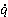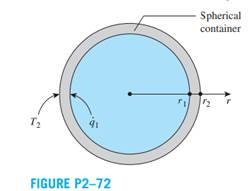### Create an Account

Home / Questions / A spherical container with an inner radius r1 1 m and an outer radius r2 105 m has its i...

# A spherical container with an inner radius r1 1 m and an outer radius r2 105 m has its inner surface subjected to a uniform heat flux of 1

A spherical container, with an inner radius r1 = 1 m and an outer radius r2 = 1.05 m, has its inner surface subjected to a uniform heat flux of1 = 7 kW/m2. The outer surface of the container has a temperature T2 = 25°C, and the container wall thermal conductivity is k = 1.5 W/m∙K. Show that the variation of temperature in the container wall can be expressed as T(r) = (1r12 /k) (1/r - 1/r2) + T2 and determine the temperature of the inner surface of the container at r = r1.Jul 21 2020 View more View LessSubscribe To Get Solution